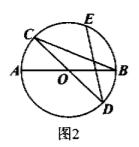$\text{A.}$ $22^{\circ}$ $\text{B.}$ $32^{\circ}$ $\text{C.}$ $34^{\circ}$ $\text{D.}$ $44^{\circ}$
【答案】 C